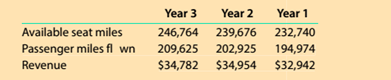Chapter 13, Problem 13.6.2MBA

Chapter
Section
Textbook Problem

Utilization rate Delta Air Lines (DAL) reported the following data (in millions) for three recent years.Delta refers to its utilization rates as passenger load factor.Compute the passenger revenue per seat mile flown. Express in cents rounded to one decimal place.

To determine

Concept Introduction:

Standard Costing System:

Standard Costing system allows estimating the costs, preparing budgets for future periods, and analyzing the performance by comparing the budgets with actual results and find variances.

To Calculate:

The Passenger Revenue per seat mile flown

Explanation

The Passenger Revenue per seat mile flown is calculated as follows:

 Year 3 Year 2 Year 1 Revenue (A) \$ 34,782 \$ 34,9...

Still sussing out bartleby?

Check out a sample textbook solution.

See a sample solution

The Solution to Your Study Problems

Bartleby provides explanations to thousands of textbook problems written by our experts, many with advanced degrees!

Get Started

Find more solutions based on key concepts